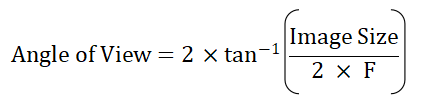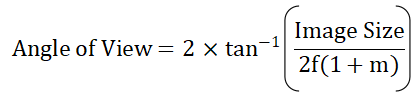# Focal Length Calculator (Thin Lens)

This is an online calculator to find the focal length of a lens. Just enter the object size, image size, and object distance to get the magnification, angle of view, and focal length value.

• mm
• mm
• mm

### Result

• Magnification
• Focal Length of the Lens
mm
• Effective Focal Length
mm
• Angle of View
Degrees

Thin Lens are those lenses whose thickness can be neglected when compared to the lengths of the radii of curvature of its two refracting surfaces, and to the distances of the objects and images from it.

Focal Length of a thin lens is the distance from the center of the lens to the principal focus of the lens.

Image formation in a lens takes place by the refraction of light at its two bounding surfaces. Each surface of the lens acts as an image-forming component and contributes to the final image formed by the lens.Consider a thin convex lens XY with an optical center at C. Let the refractive index of the lens µ2 and that of the surrounding medium be µ1. The centers of curvature of the two refracting surfaces of the lens are C1 and C2 and let the corresponding radii of curvature be R1 and R2 respectively. Consider a point object 'O' placed on the principal axis of the lens.

A ray of light OA strikes the first surface at A and is refracted in the direction 'BI1'. This ray is further refracted by the second surface in the direction of 'BI' and meets the ray which passes undeviated through the principal axis at 'I'. So, the final image is formed at 'I' after the refraction by the two surfaces of the lens.

Considering the refraction at the spherical surfaces we can derive an equation for the image formation. The basic concept is that the image formed by the first refracting surface acts as a virtual object for the second refracting surface. By applying the Gauss Formula which is a geometrical method to describe the behavior of light, we can write,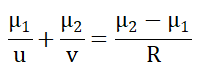The first surface XAY forms a real point-image I1. So in the above equation, v can be replaced by v1 and R by R1. Using sign convention, the distances measured to the left of the principal axis is negative. So, here u is negative and the above equation can be rewritten as,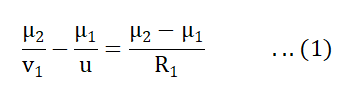From the figure, the light leaving the first surface would form an image I1 if the second surface was absent. With the second surface, I1 becomes a virtual object for the second surface and it forms an image at I, which is the final image. The equation for the final image formation can be written using the Gauss formula. Here we take u = v and R = R2, thus we get,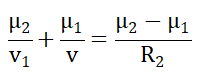Applying the sign convention, we get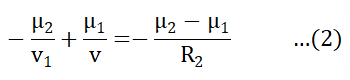Adding (1) and (2), and simplifying we get,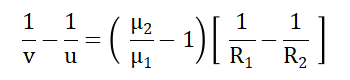Taking the surrounding medium as air, µ1=1 and also taking the refractive index of the lens as µ, we get,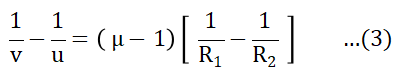If we consider the object at infinity, the image will be formed at the principal focus of the lens. So put u=∞ and v=f in the above equation and can be rewritten as,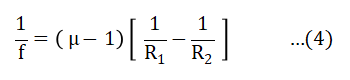This equation is known as the Lens Maker’s Formula.

Comparing equations (3) and (4), we get,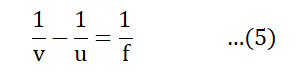This equation is the Gauss’ Formula for a lens.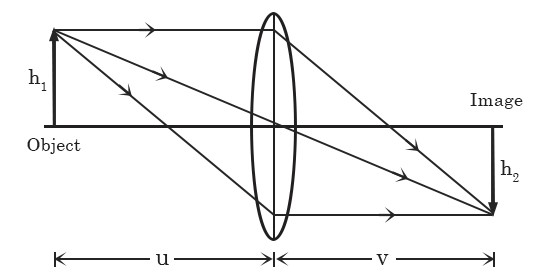Lenses are used to form a magnified image of an object. Magnification is defined as,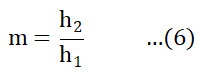where h1 and h2 are the object height and image height respectively.

By referring to figure 2, we take the refractive indices on either side of the lens as the same, then we have,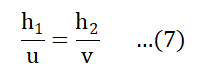So comparing (6) and (7), we get,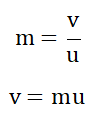Substituting this value of v in equation (5) and simplifying, we get,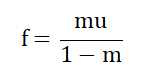In the case of a thin lens, where the lens-to-object distance is comparable to the focal length, we need to take into account the magnification factor, so we can write the effective focal length as,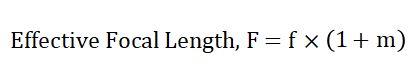Angle of View is the angular measure of how much of the area can be captured by the lens. It is defined in terms of the image size and the effective focal length,